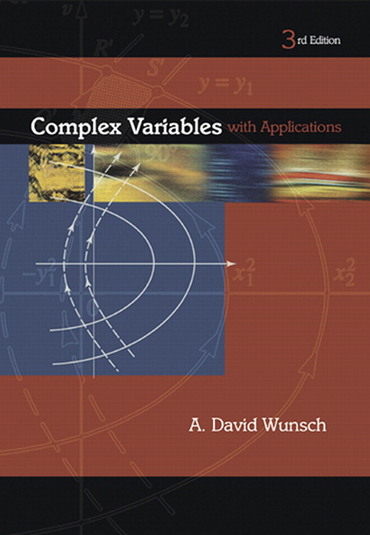# Complex Variables with Applications (3rd edition)

The third edition of this unique text remains accessible to students of engineering, physics and applied mathematics with varying mathematical backgrounds. Designed for a one or two-semester course in complex analysis, there is optional review material on elementary calculus.

• Thorough coverage of applications and motivation of theory.
• Accessible text with strong engineering applications.
• A comprehensive solutions manual is available for professors, containing worked-out solutions to every problem in the book.

75.00

Only 1 left in stock

Complex Numbers.

Introduction.
More Properties of Complex Numbers.
Complex Numbers and the Argand Place.
Integer and Fractional Powers of Complex Numbers.
Loci, Points, Sets and Regions in the Complex Plane.

The Complex Function and its Derivative.

Introduction.
Limits and Continuity.
The Complex Derivative.
The Derivative and Analyticity.
Harmonic Functions.
Some Physical Applications of Harmonic Functions.

The Basic Transcendental Functions.

The Exponential Function.
Trigonometric Functions.
Hyperbolic Functions.
The Logarithmic Function.
Analyticity of the Logarithmic Function.
Complex Functions.
Inverse Trigonometric and Hyperbolic Functions.
More on Branch Cuts and Branch Points.
Appendix: Phasors.

Integration in the Complex Plane.

Introduction to Line Integration.
Complex Line Integration.
Contour Integration and Green’s Theorem.
Path Independence, Indefinite Integrals, Fundamental Theorem of Calculus in the Complex Plane.
The Cauchy Integral Formula and it Extension.
Some Applications of the Cauchy Integral Formula.
Introduction to Dirichlet Problems – The Poisson Integral Formula for the Circle and Half Plane.
Appendix: Green’s Theorem in the Plane.

Infinite Series Involving a Complex Variable.

Introduction and Review of Real Series.
Complex Sequences and Convergence of Complex Series.
Uniform Convergence of Series.
Power Series and Taylor Series.
Techniques for Obtaining Taylor Series Expansions.
Laurent Series.
Properties of Analytic Functions Related to Taylor Series: Isolation of Zeros, Analytic Continuation, Zeta Function, Refelction.
The z transformation.
Appendix A: Fractals and the Mandelbrot Set.

Residues and Their Use in Integration.

Introduction Definition of the Residue.
Isolated Singularities.
Finding the Residue.
Evaluation of Real Integrals with Residue Calculus, I.
Evaluation of Integrals, II.
Evaluation on Integrals, III.
Integrals Involving Indented Contours.
Contour Integrations Involving Branch Points and Branch Cuts.
Residue Calculus Applied to Fourier Transform.
The Hilbert Transform.
Uniform Convergence of Integrals and the Gamma Function.
Principle of the Argument.

Laplace Transforms and Stability of Systems.

Laplace Transforms and their Inversion.
Stability – An Introduction.
The Nyquist Stability Criterion.
Laplace Transforms and Stability with Generalized Functions.

Conformal Mapping and Some of Its Applications.

Introduction.
The Conformal Property.
One-to-One Mappings and Mappings of Regions.
The Bilinear Transformation.
Conformal Mapping and Boundary Value Problems.
More on Boundary Value Problems – Streamlines as Boundaries.
Boundary Value Problems with Sources.
The Schwarz-Christoffel Transformation.
Appendix: The Stream Function and Capacitance.

Advanced Topics in Infinite Series and Products.

The Use of Residues to Sum Certain Numerical Series.
Partial Fraction Expansions of Functions with an Infinite Number of Poles.
Introduction to Infinite Products.
Expanding Functions in Infinite Products.
Weight 0.700 kg 9780201756098 2004 David A. Wunsch Pearson

## Reviews

There are no reviews yet.